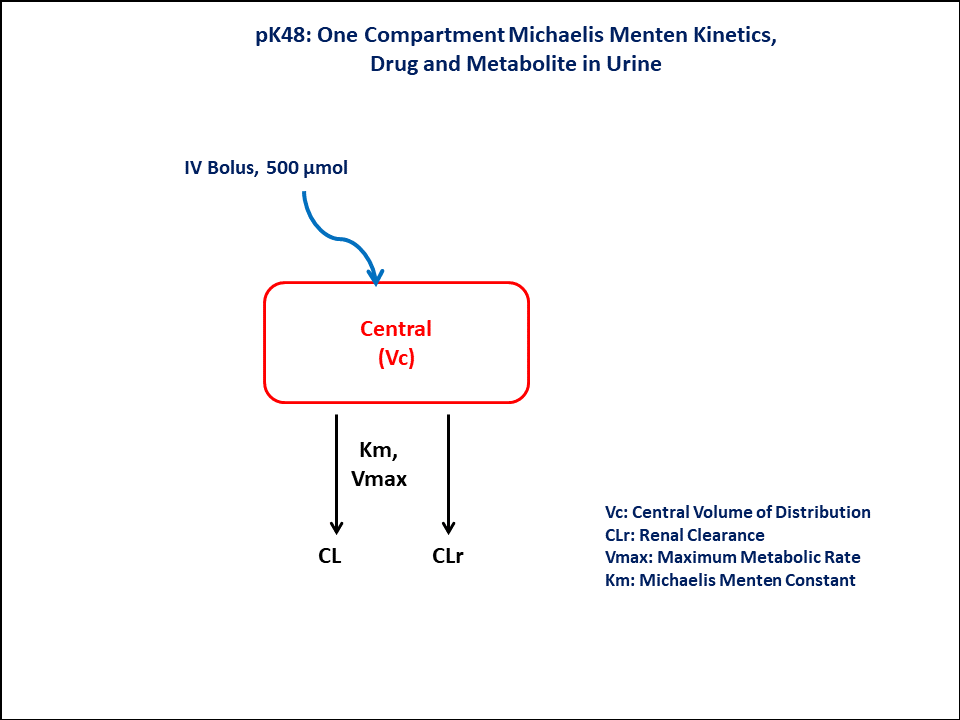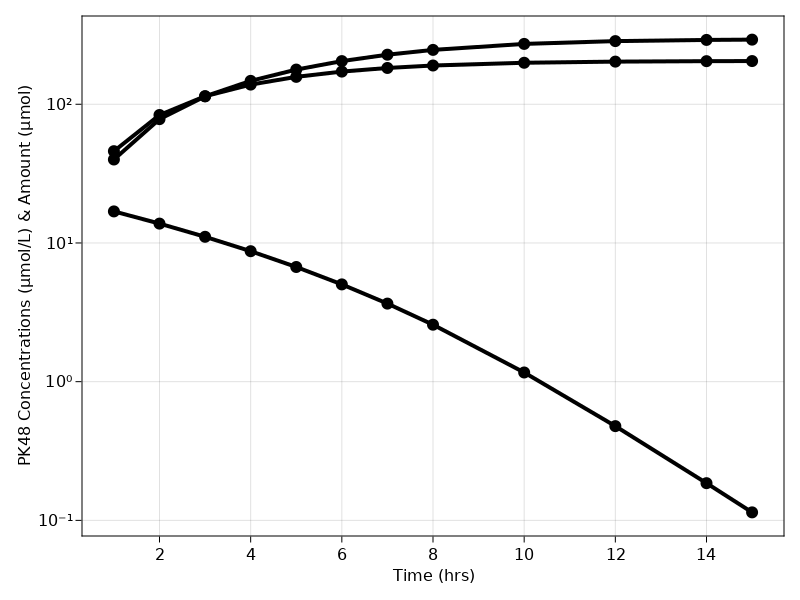# Exercise PK48 - One Compartment Michaelis Menten Kinetics, Drug and metabolite in Urine

### Background

• Structural model - One Compartment Michaelis Menten Kinetics, Drug and metabolite in Urine

• Route of administration - IV bolus

• Dosage Regimen - 500 micromol IV

• Number of Subjects - 1### Learning Outcome

In this model, you will learn -

• To build One Compartment model for the drug given Intravenous Bolus dosage, following Michaelis Menten Kinetics.

• To apply differential equation in the model as per the compartment model.

### Objectives

In this tutorial, you will learn how to build One Compartment Michaelis Menten Kinetics model, with drug and metabolite in urine

### Libraries

Call the "necessary" libraries to get started

using Random
using Pumas
using PumasUtilities
using CairoMakie
using AlgebraOfGraphics
using DataFramesMeta


### Model

In this One compartment model, we administer dose on Central compartment.

pk_48        = @model begin
desc     = "One Compartment Michaelis Menten Kinetics Model"
timeu    = u"hr"
end

@param begin
"Maximum rate of metabolism (uM/hr)"
tvvmax   ∈ RealDomain(lower=0)
"Michaelis Menten Constant (uM)"
tvkm     ∈ RealDomain(lower=0)
"Renal Clearance (L/hr)"
tvclr    ∈ RealDomain(lower=0)
"Central Volume of Distribution (L)"
tvvc     ∈ RealDomain(lower=0)
Ω        ∈ PDiagDomain(4)
"Proportional RUV"
σ²_prop  ∈ RealDomain(lower=0)
end

@random begin
η        ~ MvNormal(Ω)
end

@pre begin
Vmax     = tvvmax * exp(η)
Km       = tvkm * exp(η)
Clr      = tvclr * exp(η)
Vc       = tvvc * exp(η)
end

@vars begin
VMKM    := Vmax*(Central/Vc)/(Km + (Central/Vc))
end

@dynamics begin
Central' = -VMKM - (Clr/Vc)* Central
UrineP'  = (Clr/Vc) * Central
UrineM'  =  VMKM
end

@derived begin
cp       = @. Central/Vc
ae_p     = @. UrineP
ae_m     = @. UrineM
"""
Observed Concentration (umol/L)
"""
dv       ~ @. Normal(cp, sqrt(cp^2*σ²_prop))
"""
Observed Amount (umol)
"""
dv_aep   ~ @. Normal(ae_p, sqrt(cp^2*σ²_prop))
"""
Observed Amount (umol)
"""
dv_aem   ~ @. Normal(ae_m, sqrt(cp^2*σ²_prop))
end
end

PumasModel
Parameters: tvvmax, tvkm, tvclr, tvvc, Ω, σ²_prop
Random effects: η
Covariates:
Dynamical variables: Central, UrineP, UrineM
Derived: cp, ae_p, ae_m, dv, dv_aep, dv_aem
Observed: cp, ae_p, ae_m, dv, dv_aep, dv_aem


### Parameters

The parameters are as given below. tv represents the typical value for parameters.

• $Vmax$ - Maximum rate of metabolism (uM/hr)

• $Km$ - Michaelis Menten Constant (uM)

• $Clr$ - Renal Clearance (L/hr)

• $Vc$ - Central Volume of Distribution (L)

• $Ω$ - Between Subject Variability

• $σ$ - Residual error

param = (tvvmax    = 51.4061,
tvkm      = 5.30997,
tvclr     = 2.46764,
tvvc      = 24.5279,
Ω         = Diagonal([0.0,0.0,0.0,0.0]),
σ²_prop   = 0.02)

(tvvmax = 51.4061, tvkm = 5.30997, tvclr = 2.46764, tvvc = 24.5279, Ω = [0.
0 0.0 0.0 0.0; 0.0 0.0 0.0 0.0; 0.0 0.0 0.0 0.0; 0.0 0.0 0.0 0.0], σ²_prop
= 0.02)


### Dosage Regimen

Intravenous bolus dosing of 500 micromol to a single subject at time=0.

ev1    = DosageRegimen(500, cmt = 1, time = 0)
sub1   = Subject(id = 1, events = ev1)

Subject
ID: 1
Events: 1


### Simulation & Plot

Lets simulate for plasma concentration with the specific observation time points after IV bolus dose.

Random.seed!(123)
sim_sub1 = simobs(pk_48, sub1, param, obstimes=0.1:0.1:15)


### Visualization

@chain DataFrame(sim_sub1) begin
dropmissing!(:cp)
@rsubset :time ∈ [0,1,2,3,4,5,6,7,8,10,12,14,15]
data(_) *
mapping(:time => "Time (hrs)", [:cp, :ae_p, :ae_m]) *
visual(ScatterLines, linewidth = 4, markersize = 12)
draw(axis = (;yscale = log10, xticks = 0:2:16, ylabel = "PK48 Concentrations (μmol/L) & Amount (μmol)"))
end